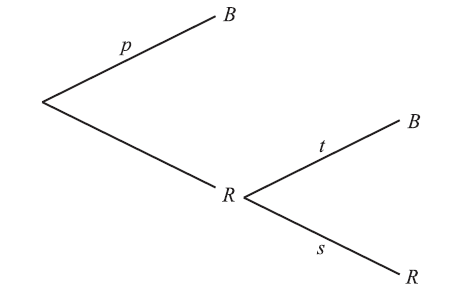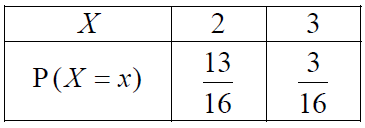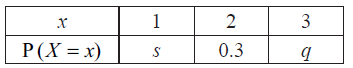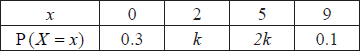# IB Math Analysis & Approaches Question bank-Topic: SL 4.7 Expected value (mean), for discrete data SL Paper 1

## Question

A four-sided die has three blue faces and one red face. The die is rolled.

Let B be the event a blue face lands down, and R be the event a red face lands down.

Write down

(i)     P(B);
(ii)    P(R).


a.

If the blue face lands down, the die is not rolled again. If the red face lands down, the die is rolled once again. This is represented by the following tree diagram, where p, s, t are probabilities.Find the value of p, of s and of t.


b.

Guiseppi plays a game where he rolls the die. If a blue face lands down, he scores 2 and is finished. If the red face lands down, he scores 1 and rolls one more time. Let X be the total score obtained.

(i)     Show that $${\text{P}}(X = 3) = \frac{3}{{16}}$$ .
(ii)    Find $${\text{P}}(X = 2)$$ .


c.

(i)     Construct a probability distribution table for X.

(ii)    Calculate the expected value of X.


d.

If the total score is 3, Guiseppi wins $$\ 10$$. If the total score is 2, Guiseppi gets nothing.

Guiseppi plays the game twice. Find the probability that he wins exactly $$\ 10$$.


e.

## Markscheme

(i) P(B) $$= \frac{3}{4}$$     A1     N1

(ii) P(R) $$= \frac{1}{4}$$     A1     N1

[2 marks]

a.

$$p = \frac{3}{4}$$     A1     N1

$$s = \frac{1}{4}$$, $$t = \frac{3}{4}$$     A1     N1

[2 marks]

b.

(i) $${\text{P}}(X = 3)$$

$$= {\text{P (getting 1 and 2)}} = \frac{1}{4} \times \frac{3}{4}$$     A1

$$= \frac{3}{{16}}$$     AG     N0

(ii) $${\text{P}}(X = 2) = \frac{1}{4} \times \frac{1}{4} + \frac{3}{4}{\text{ }}\left( {{\text{or }}1 – \frac{3}{{16}}} \right)$$     (A1)

$$= \frac{{13}}{{16}}$$      A1     N2

[3 marks]

c.

(i)A2      N2

(ii) evidence of using $${\text{E}}(X) = \sum{x{\text{P}}(X = x)}$$     (M1)
$${\text{E}}(X) = 2\left( {\frac{{13}}{{16}}} \right) + 3\left( {\frac{3}{{16}}} \right)$$    (A1)
$$= \frac{{35}}{{16}}{\text{ }}\left( { = 2\frac{3}{{16}}} \right)$$     A1     N2

[5 marks]

d.

win $$\ 10 \Rightarrow$$ scores 3 one time, 2 other time     (M1)

$${\text{P}}(3) \times {\text{P}}(2) = \frac{{13}}{{16}} \times \frac{3}{{16}}$$ (seen anywhere)     A1

evidence of recognising there are different ways of winning $$\ 10$$     (M1)
e.g. $${\text{P}}(3) \times {\text{P}}(2) + {\text{P}}(2) \times {\text{P}}(3)$$ , $$2\left( {\frac{{13}}{{16}} \times \frac{3}{{16}}} \right)$$ , $$\frac{{36}}{{256}} + \frac{3}{{256}} + \frac{{36}}{{256}} + \frac{3}{{256}}$$
$${\text{P(win }}\ 10) = \frac{{78}}{{256}}{\text{ }}\left( { = \frac{{39}}{{128}}} \right)$$     A1     N3

[4 marks]

e.

## Question

Two boxes contain numbered cards as shown below.Two cards are drawn at random, one from each box.

Copy and complete the table below to show all nine equally likely outcomes.a.

Let S be the sum of the numbers on the two cards.

Find the probability of each value of S.


b.

Find the expected value of S.


c.

Anna plays a game where she wins $$\ 50$$ if S is even and loses $$\ 30$$ if S is odd.

Anna plays the game 36 times. Find the amount she expects to have at the end of the 36 games.


d.

## MarkschemeA2     N2

[2 marks]

a.

$${\rm{P}}(12) = \frac{1}{9}$$ , $${\rm{P}}(13) = \frac{3}{9}$$ , $${\rm{P}}(14) = \frac{3}{9}$$ , $${\rm{P}}(15) = \frac{2}{9}$$     A2     N2

[2 marks]

b.

correct substitution into formula for $${\text{E}}(X)$$     A1

e.g. $${\rm{E}}(S) = 12 \times \frac{1}{9} + 13 \times \frac{3}{9} + 14 \times \frac{3}{9} + 15 \times \frac{2}{9}$$

$${\rm{E}}(S) = \frac{{123}}{9}$$     A2     N2

[3 marks]

c.

METHOD 1

correct expression for expected gain E(A) for 1 game     (A1)

e.g. $$\frac{4}{9} \times 50 – \frac{5}{9} \times 30$$

$${\rm{E}}(A) = \frac{{50}}{9}$$

amount at end = expected gain for 1 game $$\times 36$$     (M1)

= 200 (dollars)     A1     N2

METHOD 2

attempt to find expected number of wins and losses     (M1)

e.g. $$\frac{4}{9} \times 36$$ , $$\frac{5}{9} \times 36$$

attempt to find expected gain E(G)     (M1)

e.g. $$16 \times 50 – 30 \times 20$$

$${\text{E}}(G) = 200$$ (dollars)     A1     N2

[3 marks]

d.

## Question

José travels to school on a bus. On any day, the probability that José will miss the bus is $$\frac{1}{3}$$ .

If he misses his bus, the probability that he will be late for school is $$\frac{7}{8}$$ .

If he does not miss his bus, the probability that he will be late is $$\frac{3}{8}$$ .

Let E be the event “he misses his bus” and F the event “he is late for school”.

The information above is shown on the following tree diagram.Find

(i)     $${\rm{P}}(E \cap F)$$ ;

(ii)    $${\rm{P}}(F)$$ .


a(i) and (ii).

Find the probability that

(i)     José misses his bus and is not late for school;

(ii)    José missed his bus, given that he is late for school.


b(i) and (ii).

The cost for each day that José catches the bus is 3 euros. José goes to school on Monday and Tuesday.

Copy and complete the probability distribution table.c.

The cost for each day that José catches the bus is 3 euros. José goes to school on Monday and Tuesday.

Find the expected cost for José for both days.


d.

## Markscheme

(i) $$\frac{7}{{24}}$$     A1     N1

(ii) evidence of multiplying along the branches     (M1)

e.g. $$\frac{2}{3} \times \frac{5}{8}$$ , $$\frac{1}{3} \times \frac{7}{8}$$

adding probabilities of two mutually exclusive paths     (M1)

e.g. $$\left( {\frac{1}{3} \times \frac{7}{8}} \right) + \left( {\frac{2}{3} \times \frac{3}{8}} \right)$$ , $$\left( {\frac{1}{3} \times \frac{1}{8}} \right) + \left( {\frac{2}{3} \times \frac{5}{8}} \right)$$

$${\rm{P}}(F) = \frac{{13}}{{24}}$$     A1     N2

[4 marks]

a(i) and (ii).

(i) $$\frac{1}{3} \times \frac{1}{8}$$     (A1)

$$\frac{1}{{24}}$$     A1

(ii) recognizing this is $${\rm{P}}(E|F)$$     (M1)

e.g. $$\frac{7}{{24}} \div \frac{{13}}{{24}}$$

$$\frac{{168}}{{312}}$$ $$\left( { = \frac{7}{{13}}} \right)$$     A2     N3

[5 marks]

b(i) and (ii).A2A1     N3

[3 marks]

c.

correct substitution into $${\rm{E}}(X)$$ formula     (M1)

e.g. $$0 \times \frac{1}{9} + 3 \times \frac{4}{9} + 6 \times \frac{4}{9}$$ , $$\frac{{12}}{9} + \frac{{24}}{9}$$

$${\rm{E}}(X) = 4$$ (euros)     A1     N2

[2 marks]

d.

## Question

The probability distribution of a discrete random variable X is given by ${\rm{P}}(X = x) = \frac{{{x^2}}}{{14}}{\text{, }}x \in \left\{ {1{\text{, }}2{\text{, }}k} \right\}{\text{, where}} k > 0$ .

Write down $${\rm{P}}(X = 2)$$ .


a.

Show that $$k = 3$$ .


b.

Find $${\rm{E}}(X)$$ .


c.

## Markscheme

$${\rm{P}}(X = 2) = \frac{4}{{14}}$$ $$\left( { = \frac{2}{7}} \right)$$     A1     N1

[1 mark]

a.

$${\rm{P}}(X = 1) = \frac{1}{{14}}$$     (A1)

$${\rm{P}}(X = k) = \frac{{{k^2}}}{{14}}$$     (A1)

setting the sum of probabilities $$= 1$$     M1

e.g. $$\frac{1}{{14}} + \frac{4}{{14}} + \frac{{{k^2}}}{{14}} = 1$$ , $$5 + {k^2} = 14$$

$${k^2} = 9$$ (accept $$\frac{{{k^2}}}{{14}} = \frac{9}{{14}}$$ )     A1

$$k = 3$$     AG     N0

[4 marks]

b.

correct substitution into $${\rm{E}}(X) = \sum {x{\rm{P}}(X = x)}$$     A1

e.g. $$1\left( {\frac{1}{{14}}} \right) + 2\left( {\frac{4}{{14}}} \right) + 3\left( {\frac{9}{{14}}} \right)$$

$${\rm{E}}(X) = \frac{{36}}{{14}}$$ $$\left( { = \frac{{18}}{7}} \right)$$    A1     N1

[2 marks]

c.

## Question

The random variable X has the following probability distribution.Given that $${\rm{E}}(X) = 1.7$$ , find q .

## Markscheme

correct substitution into $${\rm{E}}(X) = \sum {px}$$ (seen anywhere)     A1

e.g. $$1s + 2 \times 0.3 + 3q = 1.7$$ , $$s + 3q = 1.1$$

recognizing $$\sum {p = 1}$$ (seen anywhere)     (M1)

correct substitution into $$\sum {p = 1}$$     A1

e.g. $$s + 0.3 + q = 1$$

attempt to solve simultaneous equations     (M1)

correct working     (A1)

e.g. $$0.3 + 2q = 0.7$$ , $$2s = 1$$

$$q = 0.2$$     A1     N4

[6 marks]

## Question

The random variable X has the following probability distribution, with $${\rm{P}}(X > 1) = 0.5$$ .Find the value of r .


a.

Given that $${\rm{E}}(X) = 1.4$$ , find the value of p and of q .


b.

## Markscheme

attempt to substitute $${\rm{P}}(X > 1) = 0.5$$     (M1)

e.g. $$r + 0.2 = 0.5$$

$$r = 0.3$$     A1     N2

[2 marks]

a.

correct substitution into $${\rm{E}}(X)$$ (seen anywhere)     (A1)

e.g. $$0 \times p + 1 \times q + 2 \times r + 3 \times 0.2$$

correct equation     A1

e.g. $$q + 2 \times 0.3 + 3 \times 0.2 = 1.4$$ , $$q + 1.2 = 1.4$$

$$q = 0.2$$     A1      N1

evidence of choosing $$\sum {{p_i} = 1}$$     M1

e.g. $$p + 0.2 + 0.3 + 0.2 = 1$$ , $$p + q = 0.5$$

correct working     (A1)

$$p + 0.7 = 1$$ , $$1 – 0.2 – 0.3 – 0.2$$ , $$p + 0.2 = 0.5$$

$$p = 0.3$$     A1 N2

Note: Exception to the FT rule. Award FT marks on an incorrect value of q, even if q is an inappropriate value. Do not award the final A mark for an inappropriate value of p.

[6 marks]

b.

## Question

Let $$\boldsymbol{A} = \left( {\begin{array}{*{20}{c}} 0&3 \\ { – 2}&4 \end{array}} \right)$$ and  $$\boldsymbol{B} = \left( {\begin{array}{*{20}{c}} { – 4}&0 \\ 5&1 \end{array}} \right)$$.

Find AB .


a.

Given that $${\boldsymbol{X}} – 2{\boldsymbol{A}} = {\boldsymbol{B}}$$, find X.


b.

## Markscheme

evidence of multiplying     (M1)

e.g. one correct element, $$(0 \times – 4) + (3 \times 5)$$

$${\boldsymbol{AB}} = \left( {\begin{array}{*{20}{c}} {15}&3\\ {28}&4 \end{array}} \right)$$     A2     N3

Note: Award A1 for three correct elements.

[3 marks]

a.

finding $$2{\boldsymbol{A}} = \left( {\begin{array}{*{20}{c}} 0&6\\ { – 4}&8 \end{array}} \right)$$     (A1)

adding 2$${\boldsymbol{A}}$$ to both sides (may be seen first)     (M1)

e.g. $${\boldsymbol{X}} = {\boldsymbol{B}} +2{\boldsymbol{A}}$$

$${\boldsymbol{X}} = \left( {\begin{array}{*{20}{c}} { – 4}&6\\ 1&9 \end{array}} \right)$$     A1     N2

[3 marks]

b.

## Question

The following table shows the probability distribution of a discrete random variable X .Find the value of k .


a.

Find $${\text{E}}(X)$$ .


b.

## Markscheme

evidence of summing to 1     (M1)

e.g. $$\sum\limits_{}^{} {p = 1{\text{, }}0.3 + k + 2k + 0.1 = 1}$$

correct working     (A1)

e.g. $$0.4 + 3k{\text{, }}3k = 0.6$$

$$k = 0.2$$    A1     N2

[3 marks]

a.

correct substitution into formula $${\text{E}}(X)$$      (A1)

e.g. $$0(0.3) + 2(k) + 5(2k) + 9(0.1){\text{, }}12k + 0.9$$

correct working

e.g. $$0(0.3) + 2(0.2) + 5(0.4) + 9(0.1){\text{, }}0.4 + 2.0 + 0.9$$     (A1)

$${\text{E}}(X)$$= 3.3     A1     N2

[3 marks]

b.

## Question

Jar A contains three red marbles and five green marbles. Two marbles are drawn from the jar, one after the other, without replacement.

Jar B contains six red marbles and two green marbles. A fair six-sided die is tossed. If the score is $$1$$ or $$2$$, a marble is drawn from jar A. Otherwise, a marble is drawn from jar B.

Find the probability that

(i)     none of the marbles are green;

(ii)     exactly one marble is green.


a.

Find the expected number of green marbles drawn from the jar.


b.

(i)     Write down the probability that the marble is drawn from jar B.

(ii)     Given that the marble was drawn from jar B, write down the probability that it is red.


c.

Given that the marble is red, find the probability that it was drawn from jar A.


d.

## Markscheme

(i)     attempt to find $${\rm{P(red)}} \times {\rm{P(red)}}$$     (M1)

eg   $$\frac{3}{8} \times \frac{2}{7}$$ , $$\frac{3}{8} \times \frac{3}{8}$$ , $$\frac{3}{8} \times \frac{2}{8}$$

$${\text{P(none green)}} = \frac{6}{{56}}$$ $$\left( { = \frac{3}{{28}}} \right)$$     A1     N2

(ii)     attempt to find $${\rm{P(red)}} \times {\rm{P(green)}}$$     (M1)

eg   $$\frac{5}{8} \times \frac{3}{7}$$ , $$\frac{3}{8} \times \frac{5}{8}$$ , $$\frac{{15}}{{56}}$$

recognizing two ways to get one red, one green     (M1)

eg   $$2{\rm{P}}(R) \times {\rm{P}}(G)$$ , $$\frac{5}{8} \times \frac{3}{7} + \frac{3}{8} \times \frac{5}{7}$$ , $$\frac{3}{8} \times \frac{5}{8} \times 2$$

$${\text{P(exactly one green)}} = \frac{{30}}{{56}}$$ $$\left( { = \frac{{15}}{{28}}} \right)$$     A1     N2

[5 marks]

a.

$${\text{P(both green)}} = \frac{{20}}{{56}}$$ (seen anywhere)     (A1)

correct substitution into formula for $${\rm{E}}(X)$$     A1

eg   $$0 \times \frac{6}{{56}} + 1 \times \frac{{30}}{{56}} + 2 \times \frac{{20}}{{56}}$$ , $$\frac{{30}}{{64}} + \frac{{50}}{{64}}$$

expected number of green marbles is $$\frac{{70}}{{56}}$$ $$\left( { = \frac{5}{4}} \right)$$     A1     N2

[3 marks]

b.

(i)     $${\text{P(jar B)}} = \frac{4}{6}$$ $$\left( { = \frac{2}{3}} \right)$$     A1     N1

(ii)     $${\text{P(red| jar B)}} = \frac{6}{8}$$ $$\left( { = \frac{3}{4}} \right)$$     A1     N1

[2 marks]

c.

recognizing conditional probability     (M1)

eg   $${\rm{P}}(A|R)$$ , $$\frac{{{\text{P(jar A and red)}}}}{{{\rm{P(red)}}}}$$ , tree diagram

attempt to multiply along either branch (may be seen on diagram)     (M1)

eg   $${\text{P(jar A and red)}} = \frac{1}{3} \times \frac{3}{8}$$ $$\left( { = \frac{1}{8}} \right)$$

attempt to multiply along other branch     (M1)

eg   $${\text{P(jar B and red)}} = \frac{2}{3} \times \frac{6}{8}$$ $$\left( { = \frac{1}{2}} \right)$$

adding the probabilities of two mutually exclusive paths     (A1)

eg   $${\rm{P(red)}} = \frac{1}{3} \times \frac{3}{8} + \frac{2}{3} \times \frac{6}{8}$$

correct substitution

eg   $${\text{P(jar A|red)}} = \frac{{\frac{1}{3} \times \frac{3}{8}}}{{\frac{1}{3} \times \frac{3}{8} + \frac{2}{3} \times \frac{6}{8}}}$$ , $$\frac{{\frac{1}{8}}}{{\frac{5}{8}}}$$     A1

$${\text{P(jar A|red)}} = \frac{1}{5}$$     A1     N3

[6 marks]

d.

## Question

The following diagram shows a board which is divided into three regions $$A$$, $$B$$ and $$C$$.A game consists of a contestant throwing one dart at the board. The probability of hitting each region is given in the following table.Find the probability that the dart does not hit the board.


a.

The contestant scores points as shown in the following table.Given that the game is fair, find the value of $$q$$.


b.

## Markscheme

evidence of summing probabilities to $$1$$     (M1)

eg$$\;\;\;\frac{5}{{20}} + \frac{4}{{20}} + \frac{1}{{20}} + p = 1,\;\;\;\sum { = 1}$$

correct working     (A1)

eg$$\;\;\;p = 1 – \frac{{10}}{{20}}$$

$$p = \frac{{10}}{{20}}\;\;\;\left( { = \frac{1}{2}} \right)$$     A1     N2

[3 marks]

a.

correct substitution into $${\text{E}}(X)$$     (A1)

eg$$\;\;\;\frac{4}{{20}}(q) + \frac{1}{{20}}(10) + \frac{{10}}{{20}}( – 3)$$

valid reasoning for fair game (seen anywhere, including equation)     (M1)

eg$$\;\;\;{\text{E}}(X) = 0$$, points lost$$=$$points gained

correct working     (A1)

eg$$\;\;\;4q + 10 – 30 = 0,\;\;\;\frac{4}{{20}}q + \frac{{10}}{{20}} = \frac{{30}}{{20}}$$

$$q = 5$$     A1     N2

[4 marks]

Total [7 marks]

b.

## Question

A discrete random variable $$X$$ has the following probability distribution.Find $$p$$.


a.

Find $${\text{E}}(X)$$.


b.

## Markscheme

summing probabilities to 1     (M1)

eg,$$\;\;\;\sum { = 1,{\text{ }}3 + 4 + 2 + x = 10}$$

correct working     (A1)

$$\frac{3}{{10}} + \frac{4}{{10}} + \frac{2}{{10}} + p = 1,{\text{ }}p = 1 – \frac{9}{{10}}$$

$$p = \frac{1}{{10}}$$     A1     N3

[3 marks]

a.

correct substitution into formula for $${\text{E}}(X)$$     (A1)

eg$$\;\;\;0\left( {\frac{3}{{10}}} \right) + \ldots + 3(p)$$

correct working     (A1)

eg$$\;\;\;\frac{4}{{10}} + \frac{4}{{10}} + \frac{3}{{10}}$$

$${\text{E}}(X) = \frac{{11}}{{10}}\;\;\;(1.1)$$     A1     N2

[3 marks]

Total [6 marks]

b.

## Question

Two standard six-sided dice are tossed. A diagram representing the sample space is shown below.Let $$X$$ be the sum of the scores on the two dice.

(i)     Find $${\rm{P}}(X = 6)$$ .

(ii)    Find $${\rm{P}}(X > 6)$$ .

(iii)   Find $${\rm{P}}(X = 7|X > 6)$$ .


a(i), (ii) and (iii).

Elena plays a game where she tosses two dice.

If the sum is 6, she wins 3 points.

If the sum is greater than 6, she wins 1 point.

If the sum is less than 6, she loses k points.

Find the value of k for which the game is fair.


b.

## Markscheme

(i) number of ways of getting $$X = 6$$ is 5     A1

$${\rm{P}}(X = 6) = \frac{5}{{36}}$$     A1     N2

(ii) number of ways of getting $$X > 6$$ is 21     A1

$${\rm{P}}(X > 6) = \frac{{21}}{{36}}\left( { = \frac{7}{{12}}} \right)$$     A1     N2

(iii) $${\rm{P}}(X = 7|X > 6) = \frac{6}{{21}}\left( { = \frac{2}{7}} \right)$$     A2     N2

[6 marks]

a(i), (ii) and (iii).

attempt to find $${\rm{P}}(X < 6)$$     M1

e.g. $$1 – \frac{5}{{36}} – \frac{{21}}{{36}}$$

$${\rm{P}}(X < 6) = \frac{{10}}{{36}}$$     A1

fair game if $${\rm{E}}(W) = 0$$ (may be seen anywhere)     R1

attempt to substitute into $${\rm{E}}(X)$$ formula     M1

e.g. $$3\left( {\frac{5}{{36}}} \right) + 1\left( {\frac{{21}}{{36}}} \right) – k\left( {\frac{{10}}{{36}}} \right)$$

correct substitution into $${\rm{E}}(W) = 0$$     A1

e.g. $$3\left( {\frac{5}{{36}}} \right) + 1\left( {\frac{{21}}{{36}}} \right) – k\left( {\frac{{10}}{{36}}} \right) = 0$$

work towards solving     M1

e.g. $$15 + 21 – 10k = 0$$

$$36 = 10k$$     A1

$$k = \frac{{36}}{{10}}( = 3.6)$$     A1     N4

[8 marks]

b.